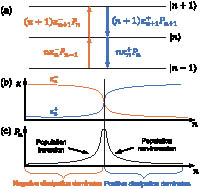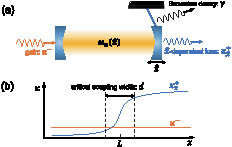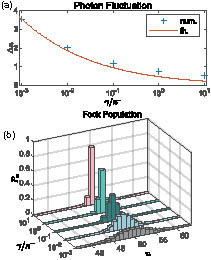Photonics Research, 2023, 11 (9): A26, Published Online: Aug. 28, 2023

### Optomechanical preparation of photon number-squeezed states with a pair of thermal reservoirs of opposite temperatures

1 State Key Laboratory of Precision Spectroscopy, Department of Physics, School of Physics and Electronic Science, East China Normal Universityhttps://ror.org/02n96ep67, Shanghai 200062, China
2 Shanghai Branch, Hefei National Laboratory, Shanghai 201315, China
3 School of Physics and Astronomy, and Tsung-Dao Lee Institute, Shanghai Jiao Tong University, Shanghai 200240, China
4 Shanghai Research Center for Quantum Sciences, Shanghai 201315, China
5 Collaborative Innovation Center of Extreme Optics, Shanxi University, Taiyuan 030006, China
Figures & Tables

#### Fig. 1. (a) Diagram of the population jump rates between neighboring Fock states. (b) Eigenvalues of the dissipation rate operators κn^± versus number n. (c) Number statistics distribution of the steady state. The probability Pn increases versus n in the region dominated by the negative-temperature dissipation and decays in the rest region dominated by the positive-temperature one, so a peak appears in the intermediate region.#### Fig. 2. (a) Cavity optomechanical scheme of feedback control. The optical cavity is coupled to the mechanical oscillator through dispersive and dissipative optomechanical interactions simultaneously. With the dispersive coupling, the oscillator undergoes a shift proportional to the radiation pressure force, i.e., to the photon number, and then changes the cavity dissipation rate κx^+ through the dissipative coupling. Except for the optomechanical dissipation, the cavity mode has a gain of rate κ− induced by the negative-temperature reservoir, and the oscillator is subjected to Brownian thermal noise. The high frequency of the optical mode makes our near-zero temperature assumption reasonable. (b) Dissipation control protocol. The positive-temperature dissipation rate κx^+ is smaller than the negative-temperature one in the region x<L but increases rapidly and overtakes it in the region x>L. The steep change occurs mainly in a region of width d.#### Fig. 3. Steady-state photon number statistics obtained by approximate solution [Eq. (13)] and numerical simulation of the master equation [Eq. (7)]. (a) Photon number fluctuation Δn versus dissipation ratio γ/κ−. The approximate solution is plotted in a red solid line, whereas, the numerical results are marked with a “+.” (b) Numerical results for the steady-state probability distribution of photon number for increasing γ/κ−. All results for g0=7.07×102ωm, (κ0,κ−,κv)=(10−1,10−2,10−3)ωm, and (d,L)=(14,7×104)xzpf.#### Table1. Experimental Parameters and Ideal Squeezing Degrees for Several Representative Optomechanical Systems

Setup$meff$ (kg)$ωm/2π$ (Hz)$g0/2π$ (Hz)$x1$ (nm)$d$ (nm)$L$ (nm)$Δn$$n¯$$Δn2/n¯$ (dB)
Micromirror $1.1×10−10$$9.7×103$22$1.27×10−8$$2.48*$50 $7×103$$4×109$−19
SiN membrane $1×10−10$$1.03×105$0.57$1×10−11$2.48100 $2.5×105$$1×1013$−22
Micro-disk $2×10−15$$2.5×107$26$2.6×10−11$0.040.02 $1.9×104$$7.7×108$−3
Levitated particle $2.8×10−18$$3×105$3 [85,86]$6.3×10−8$$0.3*$30 [87,88]$1.1×103$$4.8×108$−26
Photonic crystal $4×10−16$$4.9×106$$1.3×105$$3.5×10−6$10100$8.4×102$$2.9×107$−16
Cold atomic gases $2.4×10−22$$7×104$$3.5×106$70$25*$25000.334.8−26

Baiqiang Zhu, Keye Zhang, Weiping Zhang. Optomechanical preparation of photon number-squeezed states with a pair of thermal reservoirs of opposite temperatures[J]. Photonics Research, 2023, 11(9): A26.